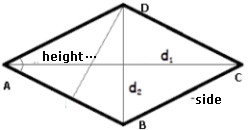### Rhombus

Rhombus is a quadrilateral, a figure of the closed form with two pairs of parallel straight segment of equal dimensions, forming equal opposite angles.
A rhombus has two diagonals, one short and one long.
See below, the important properties of a rhombus:
a) perimeter of the rhombus is equal to the sum of all sides;
b) the sum of the interior angles is always equal to 360 degrees;
c) the two diagonals bisect at right angles;
d) the diagonals have unequal lengths.
To calculate some elements of a Rhombus, enter two elements to find the others.
Use the point as a decimal separator. Ex 1,300.34 enter: 1300.34; The results will be shown after a click on CalculateRhombus Decimal places: Side Height Area Diagonal B D Diagonal A C Angles A=C Angles B=D Perimeter: Area: Angle A = C: Angle B = D: Diagonal A C: Diagonal B D: Heihgt: Side: Note:

Note:The accuracy of the calculator and its applicability to particular cases is not guaranteed.